# Modify Numpy array to store an arbitrary length string

NumPy builds on (and is a successor to) the successful Numeric array object. Its goal is to create the corner-stone for a useful environment for scientific computing. NumPy provides two fundamental objects: an N-dimensional array object (ndarray) and a universal function object (ufunc).

The dtype of any numpy array containing string values is the maximum length of any string present in the array. Once set, it will only be able to store new string having length not more than the maximum length at the time of the creation. If we try to reassign some another string value having length greater than the maximum length of the existing elements, it simply discards all the values beyond the maximum length.

In this post we are going to discuss ways in which we can overcome this problem and create a numpy array of arbitrary length.

Let’s first visualize the problem with creating an arbitrary length numpy array of string type.

 `# importing numpy as np ` `import` `numpy as np ` ` `  `# Create the numpy array ` `country ``=` `np.array([``'USA'``, ``'Japan'``, ``'UK'``, '``', '``India``', '``China']) ` ` `  `# Print the array ` `print``(country) `

Output :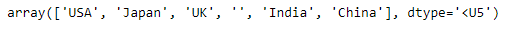As we can see in the output, the maximum length of any string length element in the given array is 5. Let’s try to assign a value having greater length at the place of missing value in the array.

 `# Assign 'New Zealand' at the place of missing value ` `country[country ``=``=` `'``'] = '``New Zealand' ` ` `  `# Print the modified array ` `print``(country) `

Output :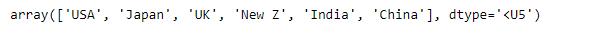As we can see in the output, ‘New Z’ has been assigned rather than ‘New Zealand’ because of the limitation to the length. Now, let’s see the ways in which we can overcome this problem.

Problem #1 : Create a numpy array of arbitrary length.

Solution : While creating the array assign the ‘object’ dtype to it. This lets you have all the behaviors of the python string.

 `# importing the numpy library as np ` `import` `numpy as np ` ` `  `# Create a numpy array ` `# set the dtype to object ` `country ``=` `np.array([``'USA'``, ``'Japan'``, ``'UK'``, '``', '``India``', '``China``'], dtype = '``object``') ` ` `  `# Print the array ` `print``(country) `

Output :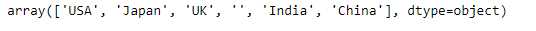Now we will use assign a value of arbitrary length at the place of missing value in the given array.

 `# Assign 'New Zealand' to the missing value ` `country[country ``=``=` `'``'] = '``New Zealand' ` ` `  `# Print the array ` `print``(country) `

Output :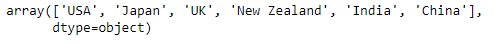As we can see in the output, we have successfully assigned an arbitrary length string to the given array object.

Problem #2 : Create a numpy array of arbitrary length.

Solution : We will use the `numpy.astype()` function to change the dtype of the given array object.

 `# importing the numpy library as np ` `import` `numpy as np ` ` `  `# Create a numpy array ` `# Notice we have not set the dtype of the object ` `# this will lead to the length problem  ` `country ``=` `np.array([``'USA'``, ``'Japan'``, ``'UK'``, '``', '``India``', '``China']) ` ` `  `# Print the array ` `print``(country) `

Output :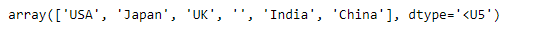Now we will change the dtype of the given array object using `numpy.astype()` function. Then we will assign an arbitrary length string to it.

 `# Change the dtype of the country ` `# object to 'U256' ` `country ``=` `country.astype(``'U256'``) ` ` `  `# Assign 'New Zealand' to the missing value ` `country[country ``=``=` `'``'] = '``New Zealand' ` ` `  `# Print the array ` `print``(country) `

Output :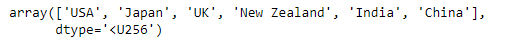As we can see in the output, we have successfully assigned an arbitrary length string to the given array object.

Note : The maximum length of the string that we can assign in this case after changing the dtype is 256.

My Personal Notes arrow_drop_upCheck out this Author's contributed articles.

If you like GeeksforGeeks and would like to contribute, you can also write an article using contribute.geeksforgeeks.org or mail your article to contribute@geeksforgeeks.org. See your article appearing on the GeeksforGeeks main page and help other Geeks.

Please Improve this article if you find anything incorrect by clicking on the "Improve Article" button below.

Article Tags :

Be the First to upvote.

Please write to us at contribute@geeksforgeeks.org to report any issue with the above content.